# Multiplying by 4 and 8 using Doubling

Multiplying by 4 and 8 using Doubling#### Doubling Strategy for Multiplying by 4• Multiplying by 4 is the same as multiplying by 2 and then by 2 again.
• This is because 4 = 2 × 2.
• Multiplying a number by 2 is known as doubling it.
• To double a number we just add it to itself.
• To multiply a number by 4, we double it and double it again.
• 5 doubled is 5 + 5, which equals 10.
• We double again to get 10 + 10, which is 20.
• 5 × 4 = 20.

#### Doubling Strategy for Multiplying by 8• Multiplying by 8 is the same as multiplying by 2, multiplying by 2 and multiplying by 2 again.
• This is because 8 = 2 × 2 × 2.
• We double a number three times to multiply it by 8.
• 5 doubled is 5 + 5, which is 10.
• We double 10 to get 10 + 10, which is 20.
• We double 20 to get 20 + 20, which is 40.
• 5 × 8 = 40.

To multiply by 4, double the number and double it again.

To multiply a number by 8, double it, double it and double it again.• We will multiply 15 by 4 using the strategy of doubling and doubling again.
• To double 15, we add 15 to itself.
• 15 + 15 = 30 and so, 15 doubled is 30.
• We now double 30 by adding it to itself.
• 30 + 30 = 60 and so, 30 doubled is 60.
• Therefore 15 multiplied by 4 equals 60.Supporting Lessons# Multiplying by 4 and 8 by Doubling

## Multiply by 4 by Doubling Twice

To multiply a number by 4, double the number and double it again.

This is known as the double, double strategy. To double a number, add it to itself.

Multiplying by 4 is the same as multiplying by 2 and then multiplying by 2 again. This is because 4 is 2 × 2.For example, to multiply 55 by 4, we double 55 and then double it again.To double a number, we add it to itself.

55 = 50 + 5 and so, we can double 50 and double 5 separately.

50 + 50 = 100 and 5 + 5 = 10.

55 + 55 = 110.We now double 110.

We can double 110 by adding it to itself. 110 + 110 = 220.

We can see that we can just multiply each digit in 110 by 2 individually to do this most easily.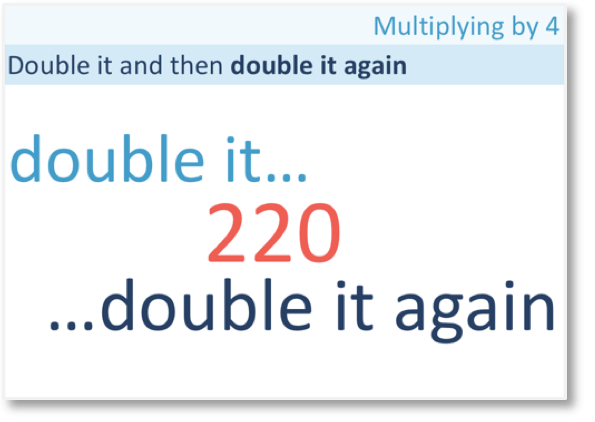We have doubled 55 twice. Therefore 55 × 4 = 220.

Because we doubled and then doubled again, this is known as the double, double strategy.Here is another example of multiplying by 4 using the double, double strategy.

Here we have 5 × 4.We double 5 by adding it to itself.

5 + 5 = 10.

We double again to complete the method,

10 + 10 = 20.

We doubled 5 twice to multiply it by 4.

5 × 4 = 20.

Here is another example of using the doubling twice method to multiply by 4.

Here we have 15 × 4.

We double 15 and then double it again to multiply it by 4.15 + 15 = 30 and so, 15 doubled is 30.

30 + 30 = 60 and so, 30 doubled is 60.

Therefore 15 × 4 = 60.

It is easy to use the doubling strategy to multiply numbers ending in 5. This is because a number ending in 5 doubled will end in 0.

When using the doubling strategy to multiply other larger numbers by 4, it is worth showing all steps.

Here is 26 × 4.

We can double 26 by doubling 20 and 6 separately and adding the results together.

20 + 20 = 40 and 6 + 6 = 12.

40 + 12 = 52 and so, 26 doubled is 52.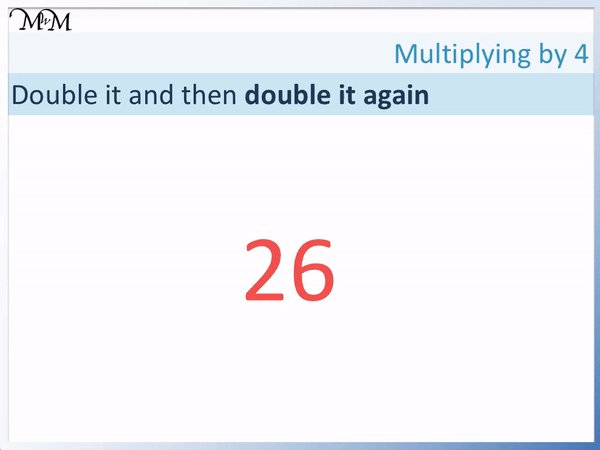We now double 52.

50 + 50 = 100 and 2 + 2 = 4.

Therefore 52 doubled is 104. Therefore 26 × 4 = 104.

The double double strategy is a useful strategy to multiply large numbers by 4.

For example, here is the number 620.

We can most easily double 620 by doubling the 6 hundreds and the 20 separately.

6 hundreds doubled is 12 hundreds. 600 doubled is 1200.

20 doubled is 40.

Therefore 620 doubled is 1240.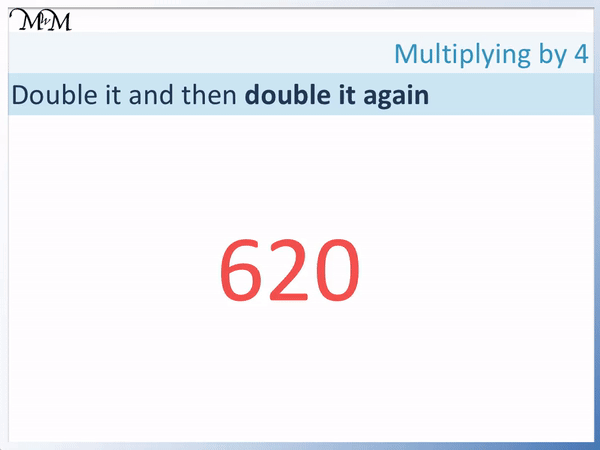We now double this again. We can double the digits 1, 2, 4 and 0 separately to get 2, 4, 8 and 0.

620 multiplied by 4 = 2480.

## Multiply by 8 using the Double, Double, Double Strategy

To multiply a number by 8, double it, double it and double it again. This is known as the double, double, double strategy.

This is because 8 = 2 × 2 × 2. To double a number, add it to itself.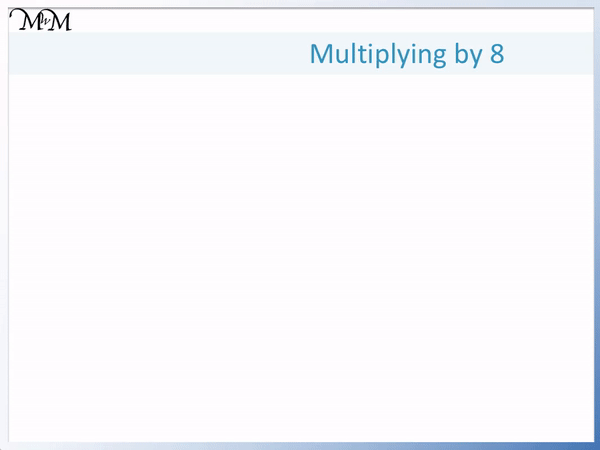For example, to multiply 54 by 8 we double it, double it and double it again.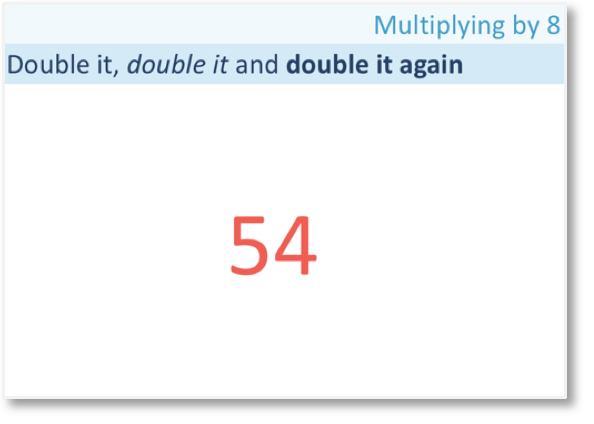To double 54, add it to itself.

50 + 50 = 100 and 4 + 4 = 8.

54 doubled equals 108.Next we double 108 by adding it to itself.

100 + 100 = 200 and 8 + 8 = 16.

108 doubled equals 216.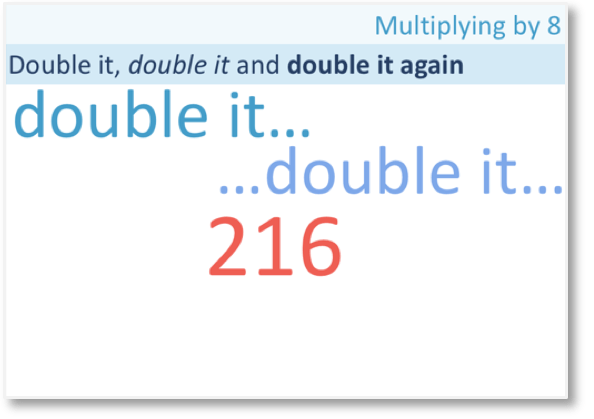We have doubled 54 twice. We need to double it again to complete the method.

200 + 200 = 400 and 16 + 16 = 32.

216 doubled equals 432.54 × 8 = 432

To multiply 54 by 8, we doubled it, doubled it and doubled it again.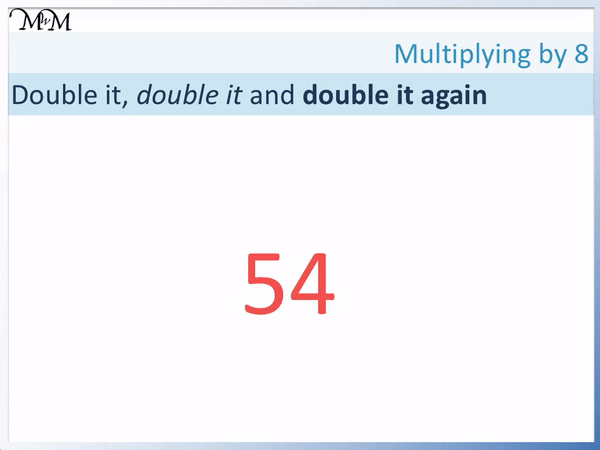Here is another example of using the double, double, double strategy to multiply by 8.

We will multiply 5 by 8 by doubling it three times.5 + 5 = 10 and so, 5 doubled is 10.

10 + 10 = 20 and so, 10 doubled is 20.

20 + 20 = 40 and so, 20 doubled is 40.

5 multiplied by 8 equals 40.

Here is another example of 12 multiplied by 8.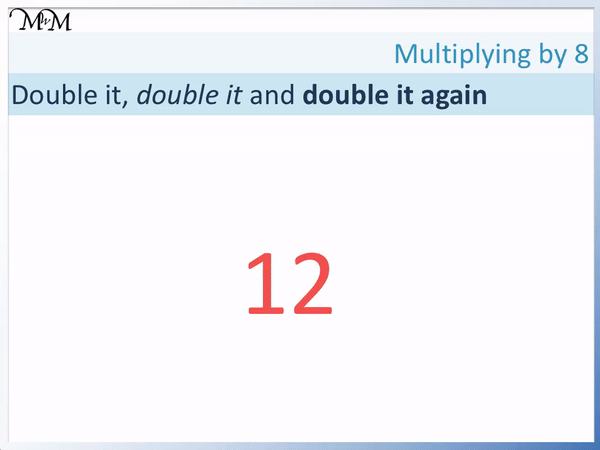12 + 12 = 24 and so, 12 doubled is 24.

24 + 24 = 48 and so, 24 doubled is 48.

To double 48, we can double 40 and 8 separately.

40 + 40 = 80 and 8 + 8 = 16.

48 doubled is 96. Here is the example of 411 multiplied by 8.

We will use the double, double, double method.

411 can be doubled by multiplying each digit in the number by 2.

411 doubled is 822.We double 822 by doubling 800 and 22 separately.

8 hundreds doubled is 16 hundred. 800 doubled is 1600.

22 doubled is 44.

Therefore 822 doubled is 1644.

We have doubled twice and we need to double 1644 once more. 1644 = 1600 + 44.

16 hundreds doubled is 32 hundreds and so, 1600 doubled is 3200.

44 doubled is 88.

1644 doubled is 3288 and so, 411 multiplied by 8 equals 3288.Now try our lesson on Multiplication Compensation Strategy where we learn how to multiply by 9 and 19.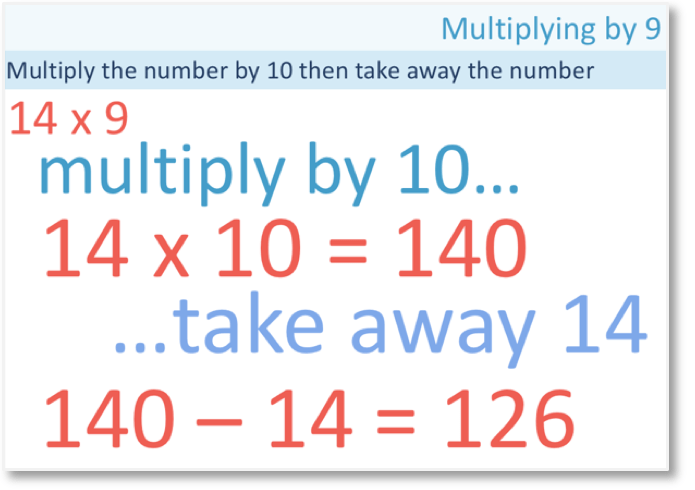error: Content is protected !!